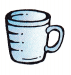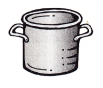# McGraw Hill Math Grade 3 Chapter 9 Lesson 4 Answer Key Volume in Liters and Milliliters

## McGraw-Hill Math Grade 3 Answer Key Chapter 9 Lesson 4 Volume in Liters and Milliliters

Estimate

Circle the better estimate for the amount each can hold.

Question 1.240 mL
240 L
A better estimate for the amount cup can hold is 240 mL.

Question 2.100 mL
10 L
A better estimate for the amount the paint bucket can hold is 100 mL.

Question 3.875 mL
5L
A better estimate for the amount spoon can hold is 875 mL.

Question 4.875 mL
5 L

A better estimate for the amount container can hold is 5 L.

Question 5.
Ask an adult to help you find a measuring cup marked with milliliter divisions. You also need a drinking glass. How many milliliters of water do you think the glass holds? Write your estimate. Fill the glass with water. Then pour the water into the measuring cup. Write the number of milliliters.
Estimate: _______ mL
Measurement: _______ mL